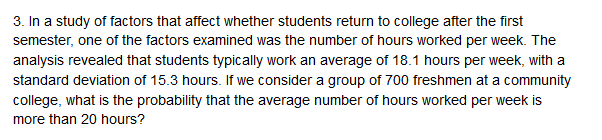3. In a study of factors that affect whether students return to college after the firstsemester, one of the factors examined was the number of hours worked per week. Theanalysis revealed that students typically work an average of 18.1 hours per week, with astandard deviation of 15.3 hours. If we consider a group of 700 freshmen at a communitycollege, what is the probability that the average number of hours worked per week ismore than 20 hours?

Questionhelp_outlineImage Transcriptionclose3. In a study of factors that affect whether students return to college after the first semester, one of the factors examined was the number of hours worked per week. The analysis revealed that students typically work an average of 18.1 hours per week, with a standard deviation of 15.3 hours. If we consider a group of 700 freshmen at a community college, what is the probability that the average number of hours worked per week is more than 20 hours? fullscreen
Step 1

Central Limit Theorem for mean:

If a random sample of size n is taken from a population having mean  and standard deviation  then, as the sample size increases, the sample mean approaches the normal distribution with mean  and standard deviation σ/ sqrt(n).

Step 2

Finding the probability that the average number of hours worked is more than 20 hours:

The students typically work an average of 18.1 hours per week. That is, μ = 18.1.

The population standard deviation is σ = 15.3.

A random sample of 700 (n) freshmen from the community college is selected.

Let the random variable X denote the number of hours worked per week. By central limit theorem for mean, the mean price of the sample follows a normal distribution with mean μ = 18.1 and standard deviation 15.3/sqrt (700) = 0.5783.Thus, the probability that the average number of hours worked per week is more than 20 hours is calculated as follows:

Step 3

Thus, the probability that the average number of hours work...

Want to see the full answer?

See Solution

Want to see this answer and more?

Our solutions are written by experts, many with advanced degrees, and available 24/7

See Solution
Tagged in

Other